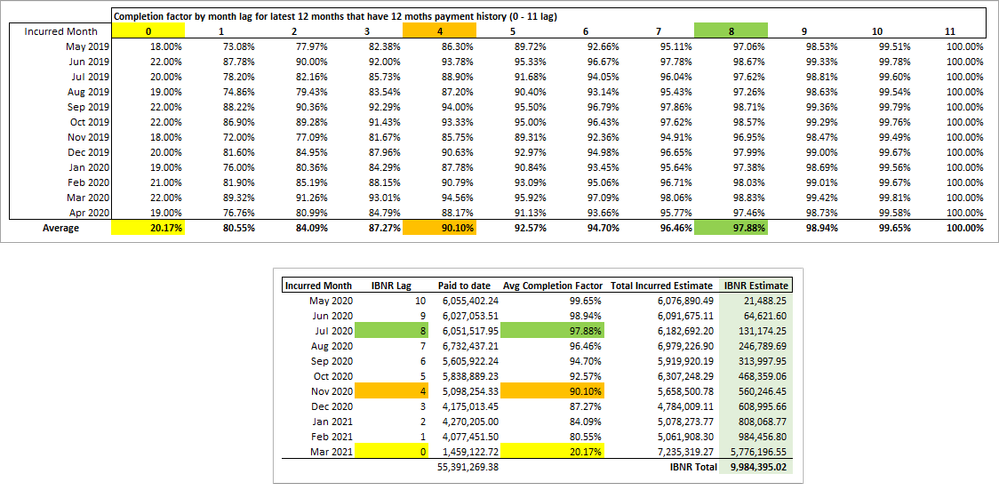cancel
Showing results for
Did you mean:Super User

## Claim completion factor and IBNR

I am working on some medical claim reporting and I am trying to get to the Incurred But Not Reported (IBNR) amount. This is an estimate of additional claim amounts still to be reported to the insurance company and is based of historical payment patterns which determines the Completion Factor.
The Completion Factor is the % of total payments that are finally made compared to the payments made through the lag month.

Lag month is the difference in months between the Incurred Date (date of service) and the Paid Date.  If a member goes to the doctor in Jan-2021 and the claim is paid in Jan-2021 that is a lag of 0.  If they go to the doctor in Jan-2021 and the claim is paid in Mar-2021 that is a lag of 2.  This can be calculated simply using DATEDIFF

``Lag = DATEDIFF ( Claims[Service Date], Claims[Paid Date], MONTH )``

What I am ultimately trying to get to is something along these lines:The IBNR is only calculated for passed months meaning, because we are in April 2021 the claims and payments data for April 2021 is not complete so any estimate would be meaningless so the first month to show is Mar 2021 and only payments posted in Mar 2021 or earlier are considered.

To calculate Completion Factors we use the payments from the most recent 12 completed months.  An incurred month is completed when it has 12 months of payment history (Lag 0 - 11) so the first completed month right now is Apr 2020 because it has all the payments from Apr 2020 through Mar 2021 posted to claims incurred in Apr 2020.  This is a general assumption that claims will be paid within 1 year of the incurred date.  These latest 12 completed months completion factor by lag is averaged and that is what is used to generate the IBNR estimate using the Paid to date amount.

• C = A / B
• D = C - A

I have attached an excel file with the above table calculated and the sample dataset also loaded into the attached .pbix.  The sample data contains 3 'Groups' which are there to help with de-bugging.

• Flat has 1000 paid every month
• Spike has most of the payments coming in the first 2 moths but the amount is the same for all lag months.
• Variable is an example of what the data might really look like with different amounts for each Incurred / Paid month pair

I simply cannot get my head around how to calcluate the avg completion factor for the complted months then use it in the IBNR estimate based on the lag and your input is greatly appreciated.2 REPLIES 2Super User

Thank you for the work you have done.  Unfortunatly the solution cannot come from a calculated table because in the real model there are other filters that can be applied to the claim table that the IBNR estimate would need to react to.

Can you think of a way that would work just using measures perhaps utilizing TREATAS on the Claim[Lag] to move the context to the 'Incurred Month'[IBNR Lag]?Community Support

I create a calculation table and calculation column, the formula is as follows:

``````Table =
SUMMARIZE(
Claims,
Claims[Group],
Claims[Lag],
'Incurred Dates'[Incurred Month]
)``````
``````Completion Factor =
DIVIDE(
CALCULATE(
SUM(Claims[Paid Amount]),
FILTER(
'Claims',
'Claims'[Lag] <= 'Table'[Lag]
&& Claims[Group] = 'Table'[Group]
&& EOMONTH(Claims[Service Date],0) = 'Table'[Incurred Month]
)
),
CALCULATE(
SUM(Claims[Paid Amount]),
FILTER(
ALLSELECTED(Claims),
Claims[Lag] <= 11
&& Claims[Group] = 'Table'[Group]
&& EOMONTH(Claims[Service Date],0) = 'Table'[Incurred Month]
)
)
)``````

After filtering the month and group, the factor obtained are as follows:Then create the following measures:

``````Avg Completion Factor =
var StartDate =
CALCULATE(
MIN('Incurred Dates'[Incurred Month]),
FILTER(
Claims,
NOT(ISBLANK([IBNR Lag]))
)
)
var EndDate =
CALCULATE(
MAX('Incurred Dates'[Incurred Month]),
FILTER(
Claims,
NOT(ISBLANK([IBNR Lag]))
)
)
return
CALCULATE(
AVERAGE('Table'[Completion Factor]),
FILTER(
'Table',
'Table'[Group] = MAX(Claims[Group])
&& 'Table'[Lag] = Claims[IBNR Lag]
&& 'Table'[Incurred Month] >= EDATE(StartDate,-12)
&& 'Table'[Incurred Month] <= EDATE(EndDate,-11)
)
)``````
``Total Incurrented Estimate = [Paid To Date]/[Avg Completion Factor]``
``````IBNR Estimate =
IF(
HASONEVALUE('Incurred Dates'[Incurred Month]),
[Total Incurrented Estimate] - [Paid To Date],
[Paid To Date]
)``````If the problem is still not resolved, please provide detailed error information or the expected result you expect. Let me know immediately, looking forward to your reply.

Best Regards,
Winniz

If this post helps, then please consider Accept it as the solution to help the other members find it more quickly.